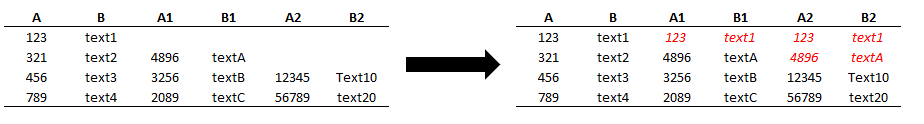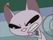cancel
Showing results for
Did you mean:Frequent Visitor

## Replace null values from multiple columns with values from other columns in place

I have a table with a level structure with some null values on upper levels and I wanted to replace those empty records with the value from the first previous level that is not null. Conceptually I thought that  using Table.TransformColumns would work if it where like:

`StepA = Table.TransformColumns(#"PreviousStep", {{"A1", each if _ = null then [A] else _}, {"A2", each if _ = null then [A1] else _},{"B1", each if _ = null then [B] else _}, {"B2", each if _ = null then [B1] else _}})`

But it shows me the error "Expression Error: We cannot apply field access to the type text", which led me to think that the function Table.TransformColumns doesn't allow me to use other columns on the expressions.I know that this could be done by creating custom columns and deleting the old ones and I realize that I could also do it with Table.ReplaceValue, but on my real scenario I have 8 levels, and those alternatives would make me have to create multiple steps, which would be pretty confusing. I would like an alternative that would allow me to solve this problem with one step overall or at max one step per level. Is it possible?

2 ACCEPTED SOLUTIONSSolution Sage

Hi, @davi

use Table.TransformRows instead. It will give you list of records in the end but you can wrap the whole step with Table.FromRows to get your table back.Frequent Visitor

Thanks @AlienSx ! I had to create one step per level like bellow and it worked:

`Table.FromRecords(Table.TransformRows(#PreviousStep,(r) => Record.TransformFields(r,{{"A1", each if _ = null then r[#"A"] else _},{"B1", each if _ = null then r[#"B"] else _}})))`
3 REPLIES 3Solution Sage

Hi, @davi

use Table.TransformRows instead. It will give you list of records in the end but you can wrap the whole step with Table.FromRows to get your table back.Frequent Visitor

Thanks @AlienSx ! I had to create one step per level like bellow and it worked:

`Table.FromRecords(Table.TransformRows(#PreviousStep,(r) => Record.TransformFields(r,{{"A1", each if _ = null then r[#"A"] else _},{"B1", each if _ = null then r[#"B"] else _}})))`Solution Sage

Neat!